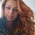Thursday, 2 March 2017

Formula based program

Formula #5 (Perimeter and area of square)

Enter length of side: 5
Perimeter of square: 20
Area of square: 25

The program for the formula is written in C# programming language and will accept a number as length. Based on the formula it will find out the perimeter and area of square.

Note: If you are new to C# and Console Application. Try to code First C# Program

Note: Read articles on how to use Loops and Conditions.

Let’s find out a simple and easy way to code these formula.

Practical Implementation:

using System;

namespace patternProblem.Formula
{
class Formula5     //perimeter and area of square
{
static void Main(string[] args)
{
Console.Write("Enter length of side: ");
double sideLength = Convert.ToDouble(Console.ReadLine());
double area = 0.0, perimeter = 0.0;

perimeter = 4 * sideLength;
area = Math.Pow(sideLength, 2);

Console.WriteLine("Perimeter of square: {0}", perimeter);
Console.WriteLine("Area of square: {0}", area);

}
}
}

Output:
The input length here is 5. So, based on the square formula. The output is shown below:

Enter length of side: 5
Perimeter of square: 20
Area of square: 25
Press any key to continue . . .

For any query, comment us below.

Skip to Main Table – Formula based program

Previous – Formula based program #4

Click imagination hunt to read latest blogs.

Keep learning and sharing...

1 comment:

1.thanks for this program! check how to learn new language in the easiest way here http://skywritingservice.com/blog/learning-a-new-language-essay

Featured post

Think that makes you rich and richer

Napolean said: “You can think and grow rich, but if you can be brought up like most people with work and you won't starve, this wil...